Next: Traveling Waves Up: Longitudinal Standing Waves Previous: Fourier Analysis

# Exercises

1. Estimate the highest possible frequency (in hertz), and the smallest possible wavelength, of a longitudinal wave in a thin Aluminium rod, due to the discrete atomic structure of this material. The mass density, Young's modulus, and atomic weight of Aluminium are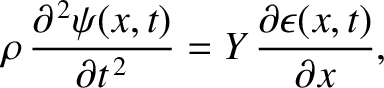,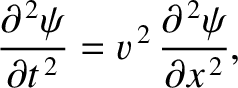, and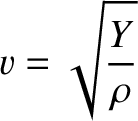, respectively. (Assume, for the sake of simplicity, that Aluminium has a simple cubic lattice.)

2. A simple model of an ionic crystal consists of a linear array of a great many equally spaced atoms of alternating masses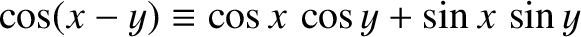and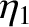, where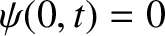. The masses are connected by identical chemical bonds that are modeled as springs of spring constant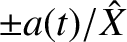. Show that the frequencies of the system's longitudinal modes of vibration either lie in the band 0 to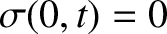or in the band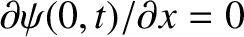to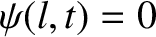. Show that, in the long wavelength limit, modes whose frequencies lie in the lower band are such that neighboring atomics move in the same direction, whereas modes whose frequencies lie in the upper band are such that neighboring atoms move in opposite directions. The lower band is known as the acoustic branch, whereas the upper band is known as the optical branch.

3. Consider a linear array of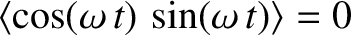identical simple pendula of massand lengththat are suspended from equal height points, evenly spaced a distance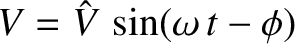apart. Suppose that each pendulum bob is attached to its two immediate neighbors by means of light springs of unstretched lengthand spring constant. The following figure shows a small part of such an array. Let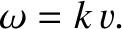be the equilibrium position of the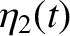th bob, for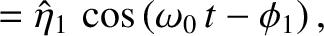, and let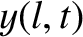be its horizontal displacement. It is assumed that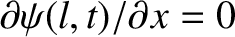for all. Demonstrate that the equation of motion of theth pendulum bob is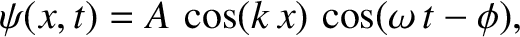Consider a general normal mode of the form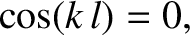Show that the associated dispersion relation is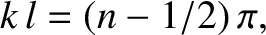Suppose that the first and last pendulums in the array are attached to immovable walls, located a horizontal distanceaway, by means of light springs of unstretched lengthand spring constant. Find the normal modes of the system. Suppose, on the other hand, that the first and last pendulums are not attached to anything on their outer sides. Find the normal modes of the system.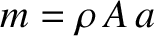4. Consider a periodic waveform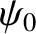of period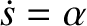, where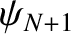for all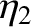, which is represented as a Fourier series,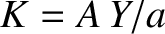where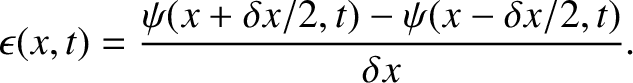. Demonstrate that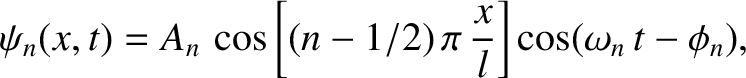where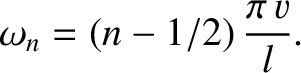and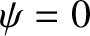, and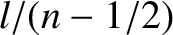where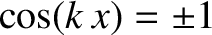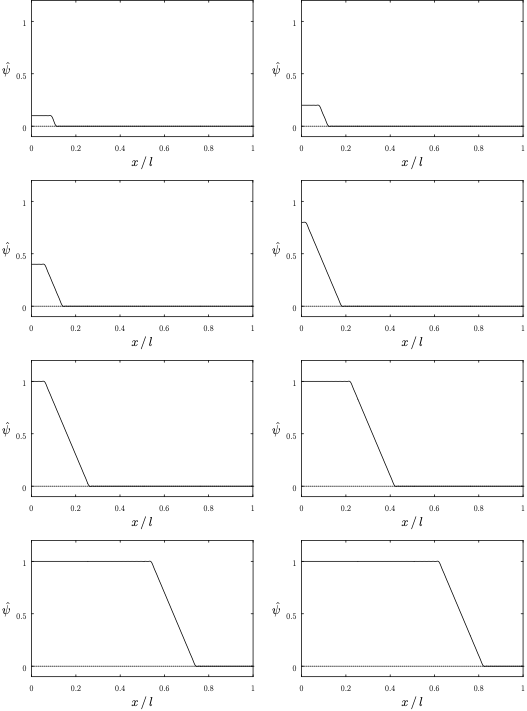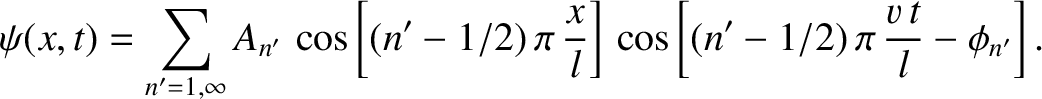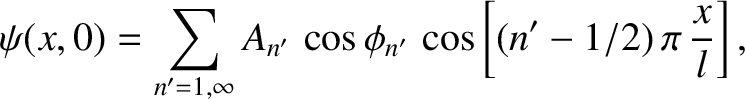5. Demonstrate that the periodic square-wave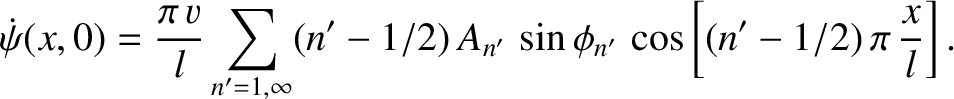wherefor all, has the Fourier representation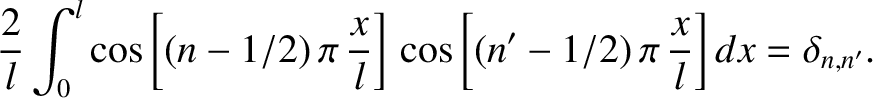Here,. Plot the reconstructed waveform, retaining the first 4, 8, 16, and 32 terms in the Fourier series.

6. Show that the periodically repeated pulse waveform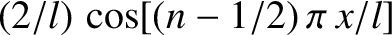where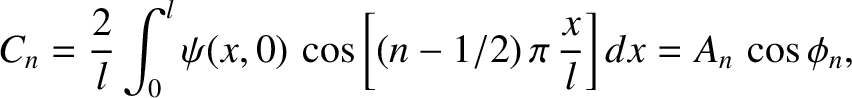for all, and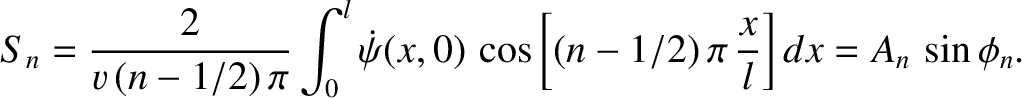, has the Fourier representationDemonstrate that if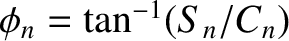then the most significant terms in the preceding series have frequencies (in hertz) that range from the fundamental frequency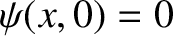to a frequency of order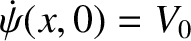.Next: Traveling Waves Up: Longitudinal Standing Waves Previous: Fourier Analysis
Richard Fitzpatrick 2013-04-08